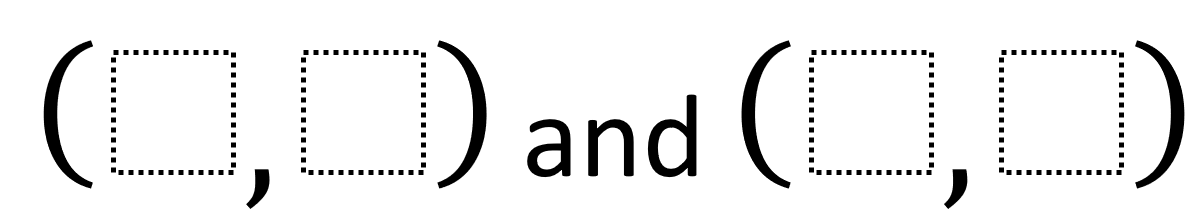# Midpoint Of A Line Segment: positive And Negative Slopes

Directions: Using the integers -9 to 9 at most one time each, place a digit in each box to create endpoints for two different line segments whose midpoint is (1, 3). One line segment should have a positive slope and the other should have a negative slope. You may reuse all the integers for each line segment.### Hint

How could you approach this problem visually? Would would a graph look like when (1, 3) is the line segment’s midpoint? What would it look like when the line segment is positive or negative? How could you approach this problem algebraically? How could you calculate to find out when the digits you’ve chosen have the correct midpoint? When you’ve found a line segment with either a positive or negative slope, how can you find the other line segment with the opposite slope?